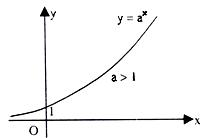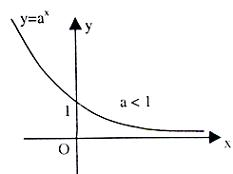×#### Thank you for registering.

One of our academic counsellors will contact you within 1 working day.

Click to Chat

1800-1023-196

+91-120-4616500

CART 0

• 0

MY CART (5)

Use Coupon: CART20 and get 20% off on all online Study Material

ITEM
DETAILS
MRP
DISCOUNT
FINAL PRICE
Total Price: Rs.

There are no items in this cart.
Continue Shopping```Exponential FunctionExponential and Logarithmic Functions

The function f(x) = ax, a > 0 where the base 'a' is constant and index x is a variable, is called an exponential function.

Clearly, x ε R so domain of f(x) is R and for no value of x, f(x) < 0 so range of 'f' is R - (-∞, 0] or (0, ∞)

Graph of an exponential function: y = ax:

The graph is different for 0 < a < 1 and a > 1, so we will discuss these cases separately.

Case I.     a > 1

Let a = 2. The domain is [-∞, ∞].

The value table is as given below

X

...

-3

-2

-1

0

1

2

3

4

5

6

...

...

f(x)

...

1/8

¼

½

1

2

4

8

16

32

64

...

...Note:

(i)     The curve approaches x-axis as x → -∞

So x-axis i.e. line y = 0 is the asymptote of y = ax. for a > 1

(ii)    This function is increasing strictly as x increases.

So, it is a strictly increasing function, hence invertible.

Case II:            0 < a < 1

Let a = 1/2  Domain of f is (-∞, ∞) The value table is as under

X

-3

-2

-1

0

1

2

3

f(x)

8

4

2

1

½

¼

1/8

We observe that(i) As x becomes very large, f(x) approaches x axis

i.e. y = 0 is the asymptote of f(x) for a < 1

(ii) y = ax decreases strictly as x increases for 0 < a < 1

So it is a strictly decreasing function. Hence, y = ax is a monotonic function for any a ≠ 1.For a < 0 the exponential function in not defined precisely and for a = 1 it turns out to be constant function.

AskIITians offers a novel way of teaching where you can prepare for IIT JEE, JEE Main /Advanced and other engineering examinations for free by sitting at home. You can visit the website askIITians.com to read the study material pertaining to your preparation. Be a part of our online tests and AQAD (A Question A Day) for free and be a winner.

To read more, Buy study materials of Set Relations and Functions comprising study notes, revision notes, video lectures, previous year solved questions etc. Also browse for more study materials on Mathematics here.
```### Course Features

• 731 Video Lectures
• Revision Notes
• Previous Year Papers
• Mind Map
• Study Planner
• NCERT Solutions
• Discussion Forum
• Test paper with Video Solution